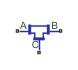# T-Junction (IL)

Three-way junction in an isothermal liquid system

• Library:
• Simscape / Fluids / Isothermal Liquid / Pipes & Fittings

•## Description

The T-Junction (IL) block models a three-way pipe junction with a branch line at port C connected at a 90o angle to the main pipe line, between ports A and B. You can specify a custom or standard junction type. When Three-way junction type is set to `Custom`, you can specify the loss coefficients of each pipe segment for converging and diverging flows. The standard model applies industry-standard loss coefficients to the momentum equations.

### Flow Direction

The flow is converging when the branch flow, the flow through port C, merges into the main flow. The flow is diverging when the branch flow splits from the main flow. The flow direction between A and I, the point where the branch meets the main, and B and I must be consistent for all loss coefficients to be applied. If they are not, as shown in the last two diagrams in the figure below, the losses in the junction are approximated with the main branch loss coefficient for converging or diverging flows.

Flow ScenariosThe block uses mode charts to determine each loss coefficient for a given flow configuration. This table describes the conditions and coefficients for each operational mode.

Flow ScenarioABCKAKBKC
Converging to node B>thresh<-ṁthresh>threshKmain,conv0Kmain,conv
Converging to node A<-ṁthresh>thresh>thresh0Kmain,convKside,conv
Diverging from node A>thresh<-ṁthresh<-ṁthresh0Kmain,divKside,div
Diverging from node B<-ṁthresh>thresh<-ṁthreshKmain,div0Kside,div
Converging to node C (branch)>thresh>thresh<-ṁthresh(Kmain,conv + Kbranch,conv)/2(Kmain,conv + Kbranch,conv)/20
Diverging from node C (branch)<-ṁthresh<-ṁthresh>thresh(Kmain,div + Kbranch,div)/2(Kmain,div + Kbranch,div)/20
Stagnant1 or last valid1 or last valid1 or last valid

The flow is stagnant when the mass flow rate conditions do not match any defined flow scenario. The mass flow rate threshold, which is the point at which the flow in the pipe begins to reverse direction, is calculated as:

`${\stackrel{˙}{m}}_{thresh}={\mathrm{Re}}_{c}\upsilon \overline{\rho }\sqrt{\frac{\pi }{4}{A}_{\mathrm{min}}},$`

where:

• Rec is the Critical Reynolds number parameter, beyond which the transitional flow regime begins.

• ν is the fluid viscosity.

• $\overline{\rho }$ is the average fluid density.

• Amin is the smallest cross-sectional area in the pipe junction.

### Standard T-Junction

When Three-way junction type is set to `Standard`, the pipe loss coefficients, Kmain and Kside, and the pipe friction factor, fT, are calculated according to Crane :

`${K}_{main,div}={K}_{main,conv}=20{f}_{T,main},$`

`${K}_{side,div}={K}_{side,conv}=60{f}_{T,side}.$`

In contrast to the custom junction type, the standard junction loss coefficient is the same for both converging and diverging flows. KA, KB, and KC are then calculated in the same manner as custom junctions.

Friction Factor per Nominal Pipe Diameter### Custom T-Junction

When Three-way junction type is set to `Custom`, the pipe loss coefficient at each port, K, is calculated based on the user-defined loss parameters for converging and diverging flow and mass flow rate at each port. You must specify Kmain,conv, Kmain,div,Kside,conv, and Kside,div as the Main branch converging loss coefficient, Main branch diverging loss coefficient, Side branch converging loss coefficient, and Side branch diverging loss coefficient parameters, respectively.

### Mass and Momentum Balance

The block conserves mass in the junction such that

`${\stackrel{˙}{m}}_{A}+{\stackrel{˙}{m}}_{B}+{\stackrel{˙}{m}}_{C}=0.$`

Flow through the pipe junction is calculated from momentum conservation equations between ports A, B, and C:

`$\begin{array}{l}{p}_{A}-{p}_{I}={I}_{A}+\frac{{K}_{A}}{2\overline{\rho }{A}_{{}_{main}}^{2}}{\stackrel{˙}{m}}_{A}\sqrt{{\stackrel{˙}{m}}_{A}^{2}+{\stackrel{˙}{m}}_{thresh}^{2}}\\ {p}_{B}-{p}_{I}={I}_{B}+\frac{{K}_{B}}{2\overline{\rho }{A}_{{}_{main}}^{2}}{\stackrel{˙}{m}}_{B}\sqrt{{\stackrel{˙}{m}}_{B}^{2}+{\stackrel{˙}{m}}_{thresh}^{2}}\\ {p}_{C}-{p}_{I}={I}_{C}+\frac{{K}_{C}}{2\overline{\rho }{A}_{{}_{side}}^{2}}{\stackrel{˙}{m}}_{C}\sqrt{{\stackrel{˙}{m}}_{C}^{2}+{\stackrel{˙}{m}}_{thresh}^{2}}\end{array}$`

where I represents the fluid inertia, and

`$\begin{array}{l}{I}_{A}={\stackrel{¨}{m}}_{A}\frac{\sqrt{\pi \cdot {A}_{side}}}{{A}_{main}}\\ {I}_{B}={\stackrel{¨}{m}}_{B}\frac{\sqrt{\pi \cdot {A}_{side}}}{{A}_{main}}\\ {I}_{C}={\stackrel{¨}{m}}_{C}\frac{\sqrt{\pi \cdot {A}_{main}}}{{A}_{side}}\end{array}$`

Amain is the Main branch area (A-B) parameter and Aside is the Side branch area (A-C, B-C) parameter.

### Energy Balance

The block balances energy such that

`${\varphi }_{A}+{\varphi }_{B}+{\varphi }_{C}=0,$`

where:

• ϕA is the energy flow rate at port A.

• ϕB is the energy flow rate at port B.

• ϕC is the energy flow rate at port C.

### Variables

To set the priority and initial target values for the block variables prior to simulation, use the Initial Targets section in the block dialog box or Property Inspector. For more information, see Set Priority and Initial Target for Block Variables.

Nominal values provide a way to specify the expected magnitude of a variable in a model. Using system scaling based on nominal values increases the simulation robustness. Nominal values can come from different sources, one of which is the Nominal Values section in the block dialog box or Property Inspector. For more information, see Modify Nominal Values for a Block Variable.

## Ports

### Conserving

expand all

Liquid entry or exit port.

Liquid entry or exit port.

Liquid entry or exit port.

## Parameters

expand all

Area of connecting pipe between ports and B.

Area of connecting pipe between ports and C and between ports B and C.

The junction loss coefficient type. Set this parameter to `Custom` to specify individual diverging and converging loss coefficients for each flow path segment.

Loss coefficient for pressure loss calculations between ports A and B for converging flow.

#### Dependencies

To enable this parameter, set Three-way junction type to `Custom`.

Loss coefficient for pressure loss calculations between ports A and B for diverging flow.

#### Dependencies

To enable this parameter, set Three-way junction type to `Custom`.

Loss coefficient for pressure loss calculations between port C and the main line for converging flow.

#### Dependencies

To enable this parameter, set Three-way junction type to `Custom`.

Loss coefficient for pressure loss calculations between port C and the main line for diverging flow.

#### Dependencies

To enable this parameter, set Three-way junction type to `Custom`.

Upper Reynolds number limit for laminar flow through the junction.

 Crane Co. Flow of Fluids Through Valves, Fittings, and Pipe TP-410. Crane Co., 1981.